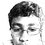# A Pattern in the Quadratic Formula!

I was going about finding the pattern of integers $$a$$ such that $$x+a=x^2$$ has at least one rational solution. Using the quadratic formula, the value boiled down to $$\frac{1\pm\sqrt{1+4a}}{2}$$. Thus, this occurs when $$4a+1$$ is the square of an integer.

I knew that odd squares can be expressed as $4a+1$, which is not very hard to prove.

Consider $y^2-1$, for some odd integer $y$. Now, this can be factorized into $(y+1)(y-1)$. Now, $2|y+1,2|y-1$, since $y$ is odd. Thus, $4|y^2-1$, or any odd $y^2$ can be expressed as $4a+1$.

Now, I was making a table of odd integers and their squares to find any patterns. Only after $25$, did I realise a pattern.

For $49$, $a=12$.

For $81$, $a=20$.

For $121$, $a=30$.

For $169$, $a=42$.

I realised a pattern, in which the difference between consecutive values of $a$ are in an arithmetic progression with difference of $2$.

I tested my theory, and found it to comply, as for $225$, $a=56$, which I predicted.

Is their a proof for this theory? Did I stumble upon some intricate pattern?Note by Nanayaranaraknas Vahdam
6 years, 3 months ago

This discussion board is a place to discuss our Daily Challenges and the math and science related to those challenges. Explanations are more than just a solution — they should explain the steps and thinking strategies that you used to obtain the solution. Comments should further the discussion of math and science.

When posting on Brilliant:

• Use the emojis to react to an explanation, whether you're congratulating a job well done , or just really confused .
• Ask specific questions about the challenge or the steps in somebody's explanation. Well-posed questions can add a lot to the discussion, but posting "I don't understand!" doesn't help anyone.
• Try to contribute something new to the discussion, whether it is an extension, generalization or other idea related to the challenge.

MarkdownAppears as
*italics* or _italics_ italics
**bold** or __bold__ bold
- bulleted- list
• bulleted
• list
1. numbered2. list
1. numbered
2. list
Note: you must add a full line of space before and after lists for them to show up correctly
paragraph 1paragraph 2

paragraph 1

paragraph 2

[example link](https://brilliant.org)example link
> This is a quote
This is a quote
    # I indented these lines
# 4 spaces, and now they show
# up as a code block.

print "hello world"
# I indented these lines
# 4 spaces, and now they show
# up as a code block.

print "hello world"
MathAppears as
Remember to wrap math in $$ ... $$ or $ ... $ to ensure proper formatting.
2 \times 3 $2 \times 3$
2^{34} $2^{34}$
a_{i-1} $a_{i-1}$
\frac{2}{3} $\frac{2}{3}$
\sqrt{2} $\sqrt{2}$
\sum_{i=1}^3 $\sum_{i=1}^3$
\sin \theta $\sin \theta$
\boxed{123} $\boxed{123}$

Sort by:

It's pretty easy to see that the difference of two consecutive a's is 2 by using that y must be odd and writing it as 2k-1.

- 6 years, 3 months ago

From the above question, $a = x^{2} - x = x(x-1)$. The examples you're interested in positive integers, that will be the common pattern of $2,6,12,20,30,42,...$

- 6 years, 3 months ago